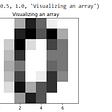# Matplotlib Tutorial-Part1

In this blog today, I will be going through the visualization tool that I used the most in python, the Matplotlib library. Matplotlib is a 2d graphic visualization library for python. It is the most commonly used library and also acts as a base for several more advanced visualization libraries.

Matploitlib is used for plotting, it provides objected-oriented APIs for integrating graphs and charts into applications.

Matplotlib is not a library that is installed by default when Python is installed. It has to be installed before it can be imported.

Next, I will go through the different types of charts and plots that are available including Bar Graph, Scatter Plot, Pie Plot, and Histogram.

To import the library we use the code below, plt is a common naming convention for matplotlib.

`from matplotlib import pyplot as plt#Plotting a simple graphplt.plot([1,4,6],[3,5,2])plt.show()# Output -`

By default, the plt.plot plotted a line chart. Next, I will plot out a graph with labels for the x and y-axis, a title, and different styling.

`from matplotlib import pyplot as pltfrom matplotlib import stylestyle.use(‘ggplot’)x = [2,4,10]y = [11,14,5]x2 = [3,4,14]y2 = [8,14,8]plt.plot(x,y,’g’,label=’line 1', linewidth=4)plt.plot(x2,y2,’c’,label=’line 2',linewidth=4)plt.title(‘Test Graph’)plt.ylabel(‘Y axis’)plt.xlabel(‘X axis’)plt.legend()plt.grid(True,color=’k’)plt.show()# Output-`

In the above graph, I changed the width of the lines and the color of each line. I also turned on the grid on the canvas behind and specified the color for it.

Next, I will talk about a few different kinds of plots and when they should be used. First is the Bar Graph.

## Matplotlib Bar Graph

So when would we need a bar graph? Bar graphs are used to compare different groups or to track changes over a certain amount of time. It is not used to plot data that continuously change over time, they are very helpful when comparing information that is collected by counting the number of entries. They should not be used if you are looking for trends over time. The bar on the graphs could be represented either horizontally or vertically. The longer the bar is the greater it is. Below is the code to implement bar graphs in matplotlib

`from matplotlib import pyplot as pltplt.bar([0.25,1.25,2.25,3.25,4.25],[2,3,1,4,5],label="Tom", color='firebrick', width=.5)plt.bar([.75,1.75,2.75,3.75,4.75],[1,2,4,6,5],label="Jane", color='indigo',width=.5)plt.legend(loc='upper left')plt.xlabel('Days')plt.ylabel('Number Of Ice Cream Bars Eaten')plt.title('Sweet Tooth')plt.show()#Output -`

Above I have plotted out a comparison between how many ice creams bars have been eaten by Tom and Jane over a period of 5 days. I specified colors for Tom and Jane as firebrick and indigo. I also put the legend box in the upper left corner since it was blocking the bars in the upper right corner.

--

--

--

## More from Htoo Latt

Love podcasts or audiobooks? Learn on the go with our new app.

## Data, Data Everywhere Nor Any Drop of Think## Robust Validation Approach for Imbalanced Data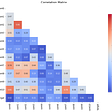## Contribution of gravity models in a business expansion plan## Artificial Intelligence Helps Distinguish the Forests from the Trees: Part I## Working With Time Series Using SQL## Let’s the clustering save your day !## The First Three Things I Do When Analysing A Time Series## 3 Ways a Deep Understanding of Bayes Theorem Will Improve Your Data Science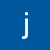## Trigonometry Topics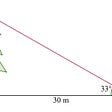## A Cyborg inquiry into destressing with drinks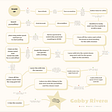## Gameplay Journal Entry #2## Recognizing Handwritten Digits with Scikit-Learn